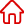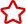﻿ 對於JSP中表單數據存儲的一種通用方法_關於JSP設為首頁 加入收藏

C語言|JAVA編程
Python編程

ASP編程|PHP編程
JSP編程

MYSQL數據庫|SqlServer數據庫
Oracle數據庫|DB2數據庫
程式師世界 >> 編程語言 >> 網頁編程 >> JSP編程 >> 關於JSP >> 對於JSP中表單數據存儲的一種通用方法

# 對於JSP中表單數據存儲的一種通用方法

J2EE(Java 2 Enterprise Edition)技術已廣泛應用在Web應用開發中，其中的JavaBean、Servlet技術為開發者提供了更為清晰的開發環境，使用JSP技術表現頁面，使用Servlet技術完成大量的業務處理，使用Bean來存儲數據及一些業務處理。在WEB應用中，業務數據存儲到數據庫中的處理工作經常很繁重，其中一種主要的形式就是表單數據存儲到數據庫，整個應用處理過程牽涉到大量的這種數據存儲操作，對每個表單都要單獨編寫相應的數據存儲程序，花費了開發人員大量的時間和精力。采用什麼方法來減輕表單數據存儲的開發工作量是值得研究的問題。

1、對每一表單都編寫相應的程序代碼

2、對每一數據表自動生成一個JavaBean程序代碼

＜jsp：useBean id="table" class="table1_bean" /＞
＜jsp：setProperty name="table" property="*" /＞
(注：table1_bean為上述自動生成的對應某一個表的JavaBean)

＜%boolean success =table.insert()； %＞

1、調用格式

＜Form Name=Frm1 Method=Post Action="DBdataStore.jsp? tablename=table1&OperType=…"＞

table1為數據將要存儲的數據庫表的表名，OperType操作類型分為三種：insert，update，delete。

2、以oracle為例的視圖定義

1) 建立表各列數據類型視圖

CREATE OR REPLACE VIEW v_dbstru
AS
SELECT table_name，column_name，data_type，data_length，
data_precision，data_scale，column_id FROM all_tab_columns
WHERE owner=user1；
//user1為數據表的屬主

2) 建立表的關鍵列視圖

CREATE OR REPLACE VIEW v_pkey_column
AS
SELECT b.table_name，b.column_name，b.position
FROM all_constraints a，all_cons_columns b
WHERE a.owner=b.owner AND a.constraint_name=b.constraint_name
AND a.owner=user1 AND a.constraint_type=P；

3、主要程序代碼

1) 程序初始化

String tablename=request.getParameter("tablename")；//提取表名
String OperType=request.getParameter("OperType")；//提取操作類型
String sFieldValue=""；//存放表單提交的字段數據值
String fieldname=""，Datatype="" //存放字段名，字段數據類型
int iFieldvalue=0；
opSql=""，strSql =""；ResultSet rs1=null，rs2=null；
insSql1="insert into "+tablename+" ("；
insSql2="values("；

2)生成sql語句關鍵字段部分

rs1=Stmt.executeQuery(
"SELECT column_name FROM v_pkey_column  WHERE table_name="+tablename+"")；
//取關鍵字段字段名while(rs1.next()){
fieldname=rs1.getString("column_name")；
rs2=Stmt.executeQuery("SELECT data_type FROM v_dbstru WHERE
table_name="+tablename+" AND column_name="+fieldname+"")；
//取關鍵字段數據類型
if(rs2.next()){
Datatype=rs2.getString("data_type")；
sFieldValue=request.getParameter(fieldname.toLowerCase())；
//生成insert語句關鍵字段部分
if(OperType.equals("insert")){
insSql1+=fieldname+"，"；
if((sFieldValue==null) ){
//表單未提交關鍵字段數據值時，本文只按數字型處理，數據值按下一流水號計算。
rs2= Stmt. executeQuery("SELECT max("+fieldname+")+1 FROM "+tablename)；
rs2. next()；
iFieldvalue=rs2.getInt(1)；
insSql2+=Integer.toString(iFieldvalue)+"，"；
}
else if(Datatype.equals("DATE")){
insSql2+= "To_Date(" + sFieldValue + "，YYYY-MM-DD)，"；
}else if(Datatype.equals("VARCHAR2") || Datatype.equals("CHAR")){
insSql2+="" + sFieldValue+"，"；
}
else /*NUMBER，FLOAT */ insSql2+=sFieldValue+"，"；}
//生成update，delete語句where部分：where fieldname=... AND
if(OperType.equals("update") || OperType.equals("delete")){
if(Datatype.equals("DATE")){
whereSql+=fieldname+"=To_Date(" + sFieldValue + "，YYYY-MM-DD) AND "；
}
else if(Datatype.equals("VARCHAR2") || Datatype.equals("CHAR")){
whereSql+=fieldname+"=" + sFieldValue+" AND "；
}else /*NUMBER，FLOAT */ whereSql+=fieldname+"="+ sFieldValue+" AND "；} }
}
whereSql=whereSql.substring(0，whereSql.length()-4)；

3)非關鍵字段部分sql語句生成

update語句，如：update table1 set column1=value1，… where id=100

insert語句，如：insert into table1(id，column1，…)values(100，value1，…)

strSql="SELECT column_name，data_type，data_length，data_precision，data_scale
FROM 　v_dbstru a "+"where table_name="+tablename+" AND a.column_name
not in (SELECT 　b.column_name FROM v_pkey_column b
where b.table_name=a.table_name)"；rs1=Stmt.executeQuery(strSql)；
//取非關鍵字段字段名和數據類型
while(rs1.next()){
fieldname=rs1.getString("column_name")；
Datatype=rs1.getString("data_type")；
sFieldValue=request.getParameter(fieldname.toLowerCase())；
//若表單未提交該字段的值，則忽略該字段的處理
if((sFieldValue!=null)){
//生成insert語句=insSql1+insSql2 即insert into tablename(… 和 values(…
if(OperType.equals("insert")){
insSql1+=